RNotes | gplots

``library(gplots)``
``````##
## Attaching package: 'gplots'
##
## The following object is masked from 'package:stats':
##
##     lowess``````
``````data(mtcars)
x  <- as.matrix(mtcars)
rc <- rainbow(nrow(x), start=0, end=.3)
cc <- rainbow(ncol(x), start=0, end=.3)``````

## demonstrate the effect of row and column dendrogram options

``heatmap.2(x)  ## default - dendrogram plotted and reordering done.``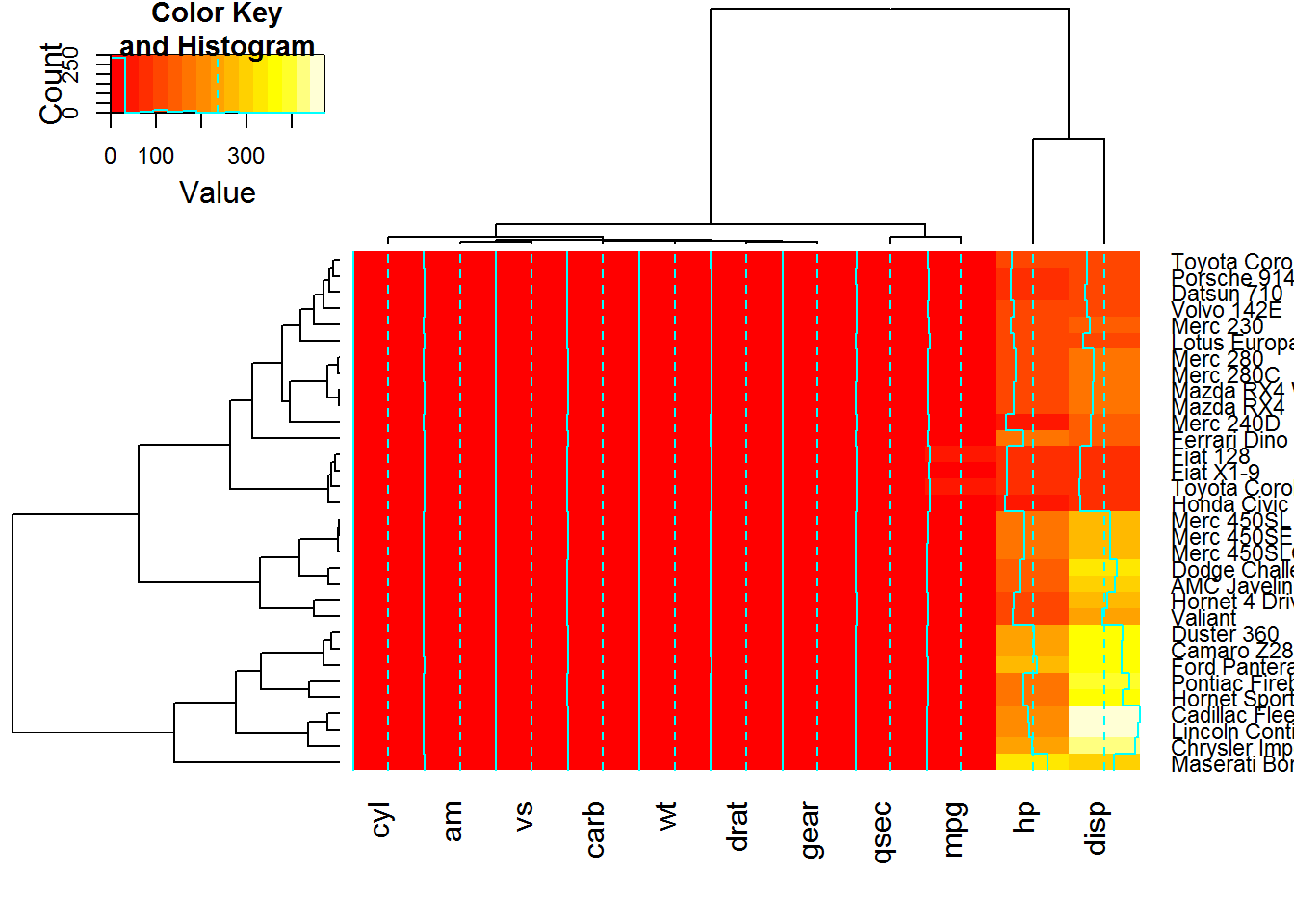``heatmap.2(x, dendrogram="none") ##  no dendrogram plotted, but reordering done.``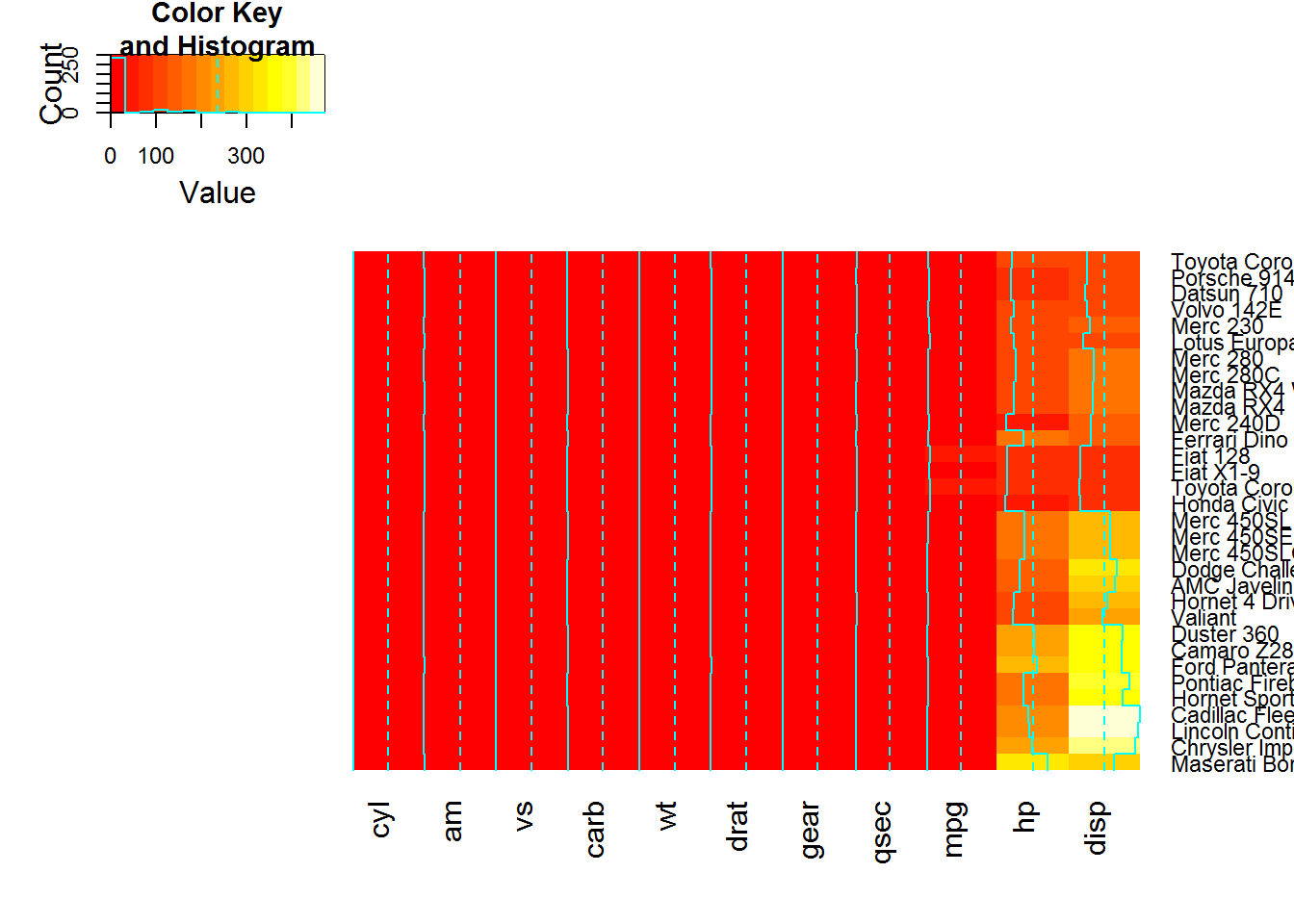``heatmap.2(x, dendrogram="row") ## row dendrogram plotted and row reordering done.``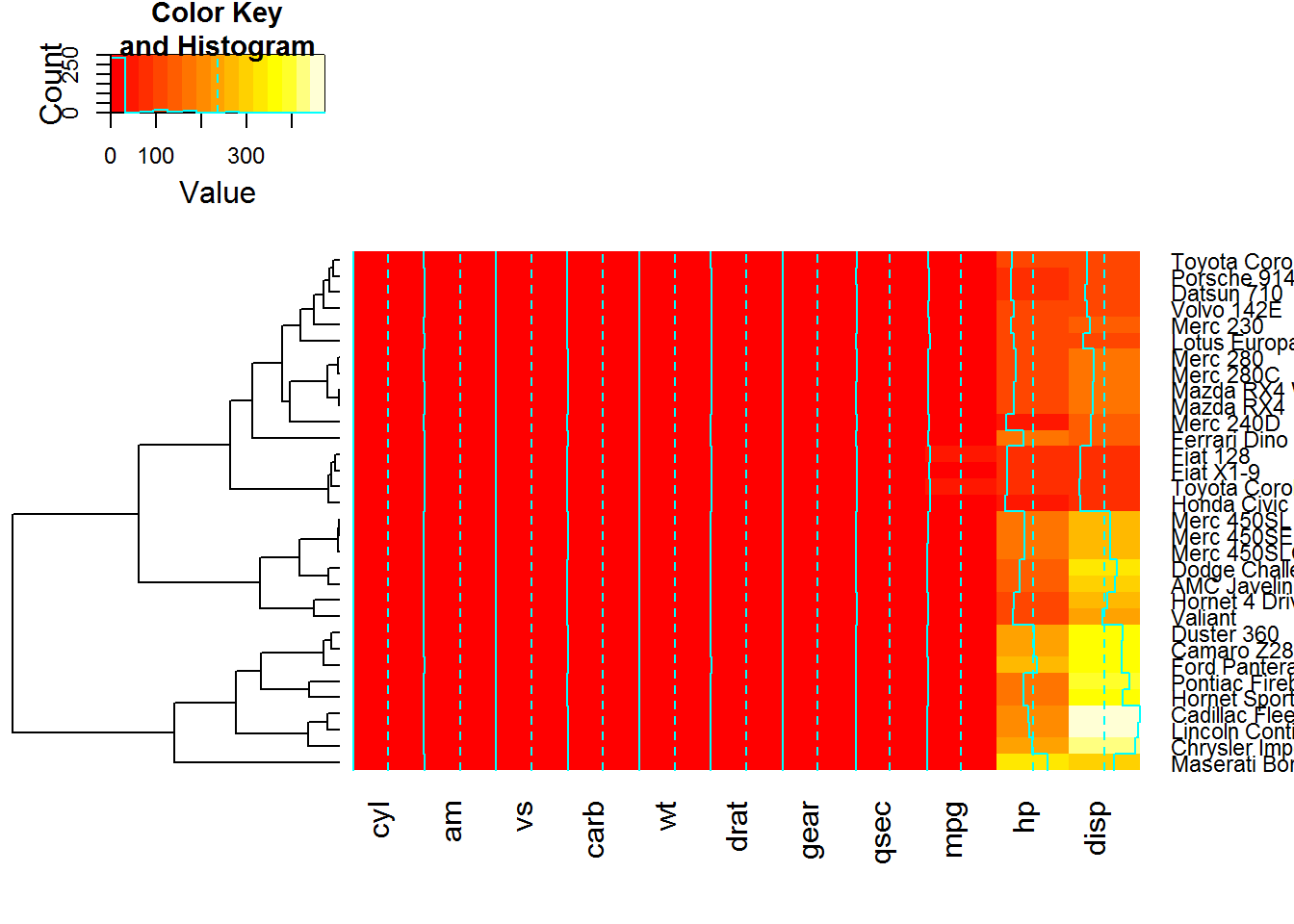``heatmap.2(x, dendrogram="col") ## col dendrogram plotted and col reordering done.``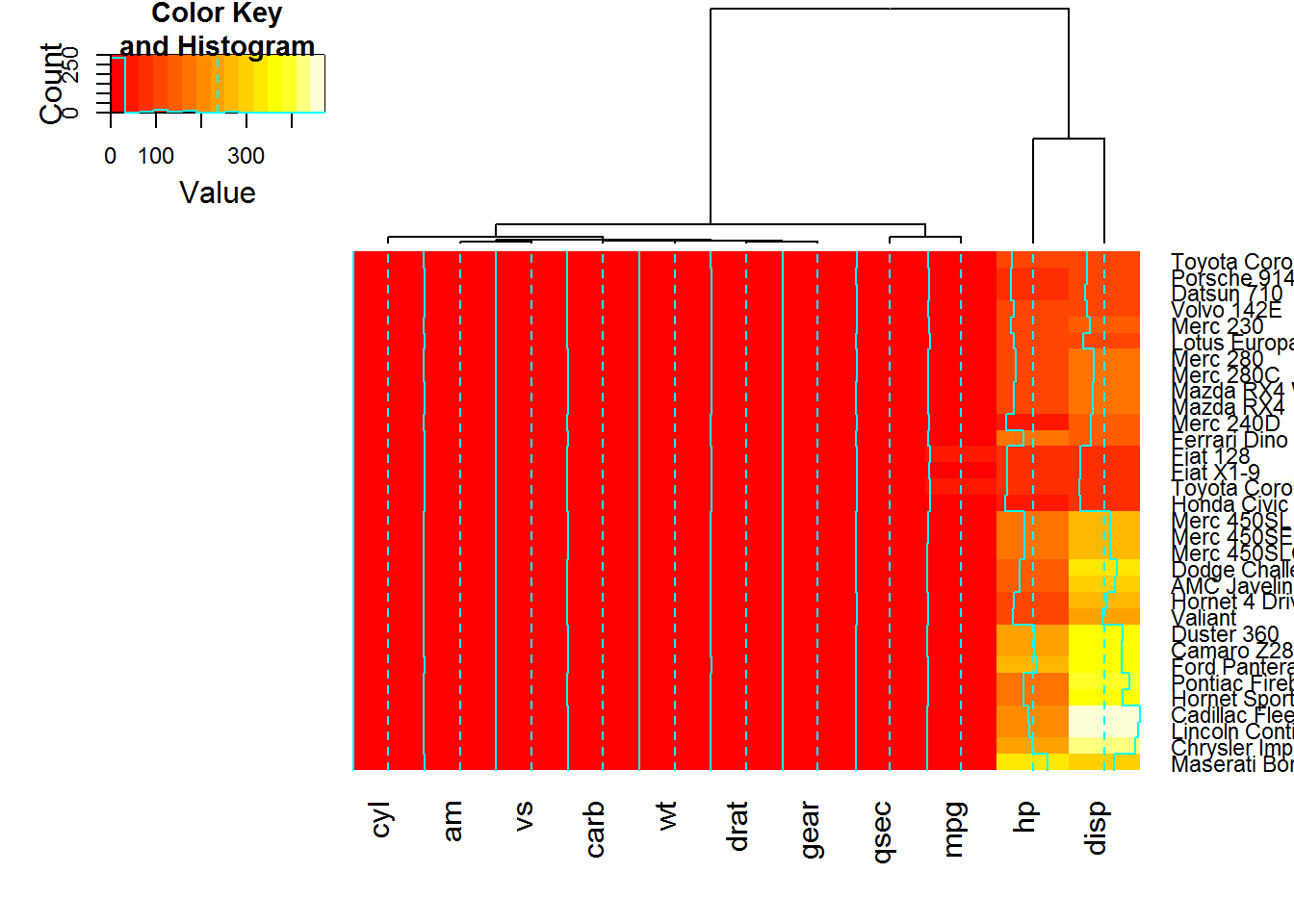``heatmap.2(x, keysize=2)  ## default - dendrogram plotted and reordering done.``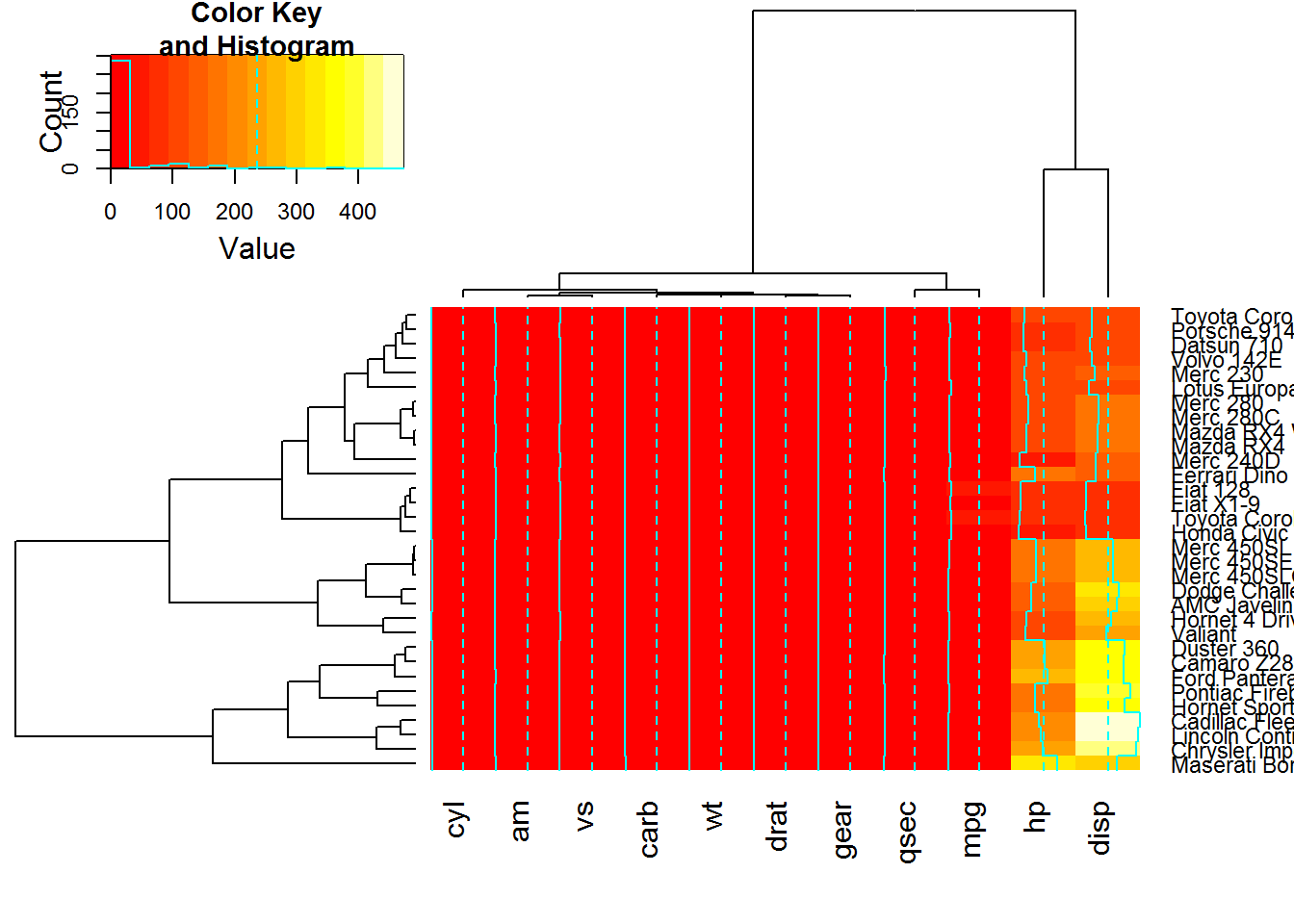``heatmap.2(x, Rowv=FALSE, dendrogram="both") ## generate warning!``
``````## Warning in heatmap.2(x, Rowv = FALSE, dendrogram = "both"): Discrepancy:
## Rowv is FALSE, while dendrogram is `column'. Omitting row dendogram.``````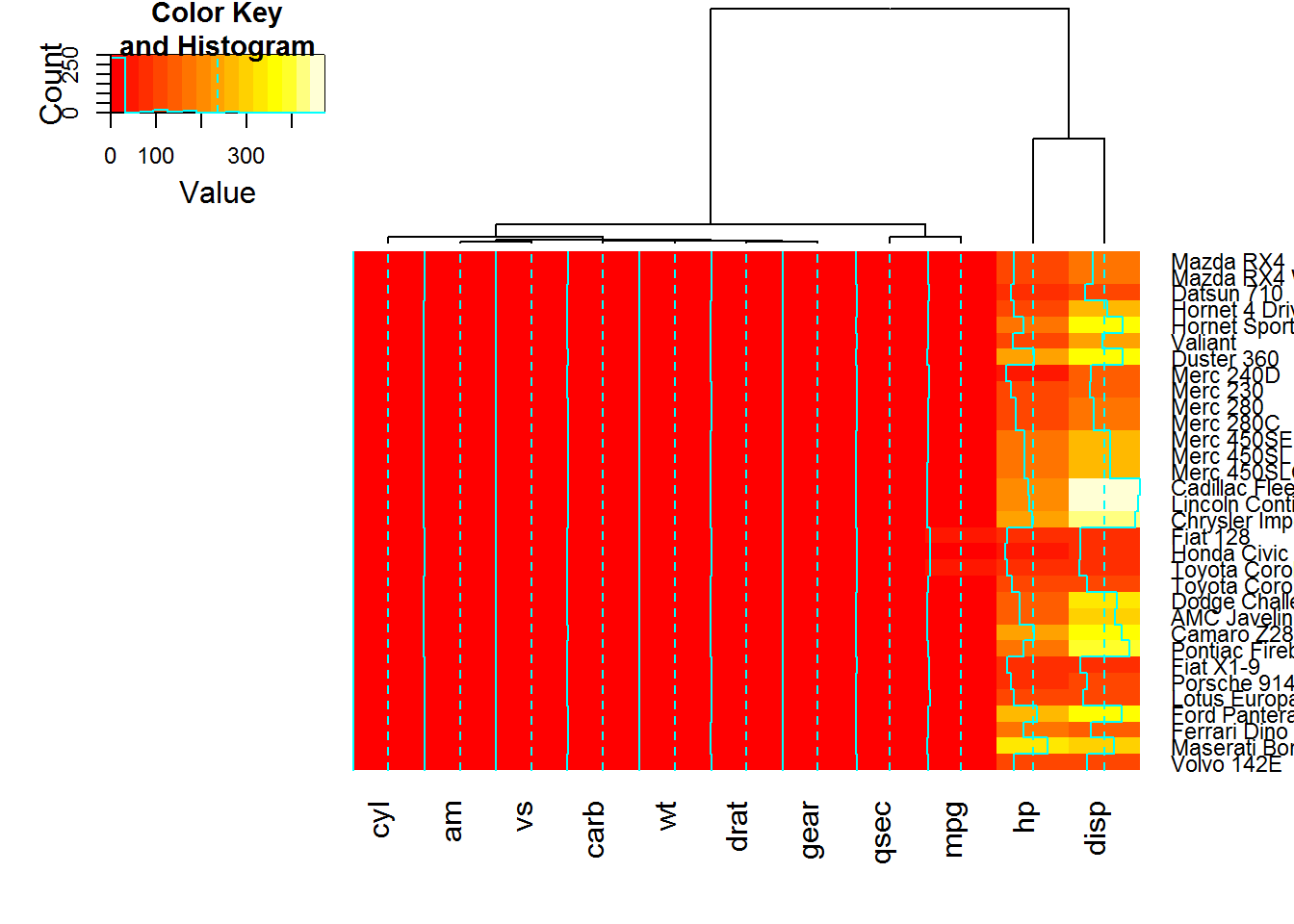``heatmap.2(x, Rowv=NULL, dendrogram="both")  ## generate warning!``
``````## Warning in heatmap.2(x, Rowv = NULL, dendrogram = "both"): Discrepancy:
## Rowv is FALSE, while dendrogram is `column'. Omitting row dendogram.``````
``heatmap.2(x, Colv=FALSE, dendrogram="both") ## generate warning!``
``````## Warning in heatmap.2(x, Colv = FALSE, dendrogram = "both"): Discrepancy:
## Colv is FALSE, while dendrogram is `row'. Omitting column dendogram.``````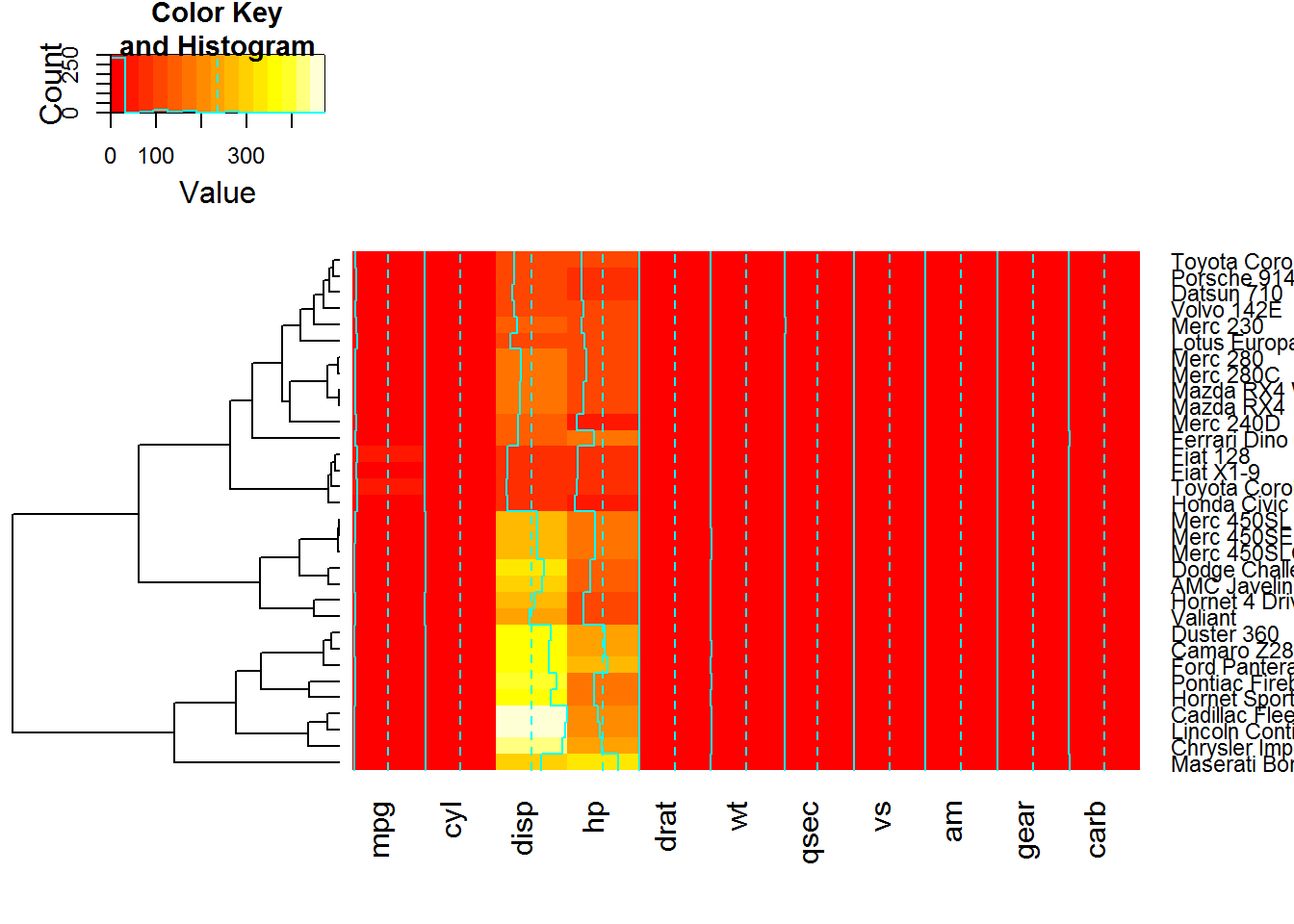``````## Reorder dendrogram by branch means rather than sums
heatmap.2(x, reorderfun=function(d, w) reorder(d, w, agglo.FUN = mean) )``````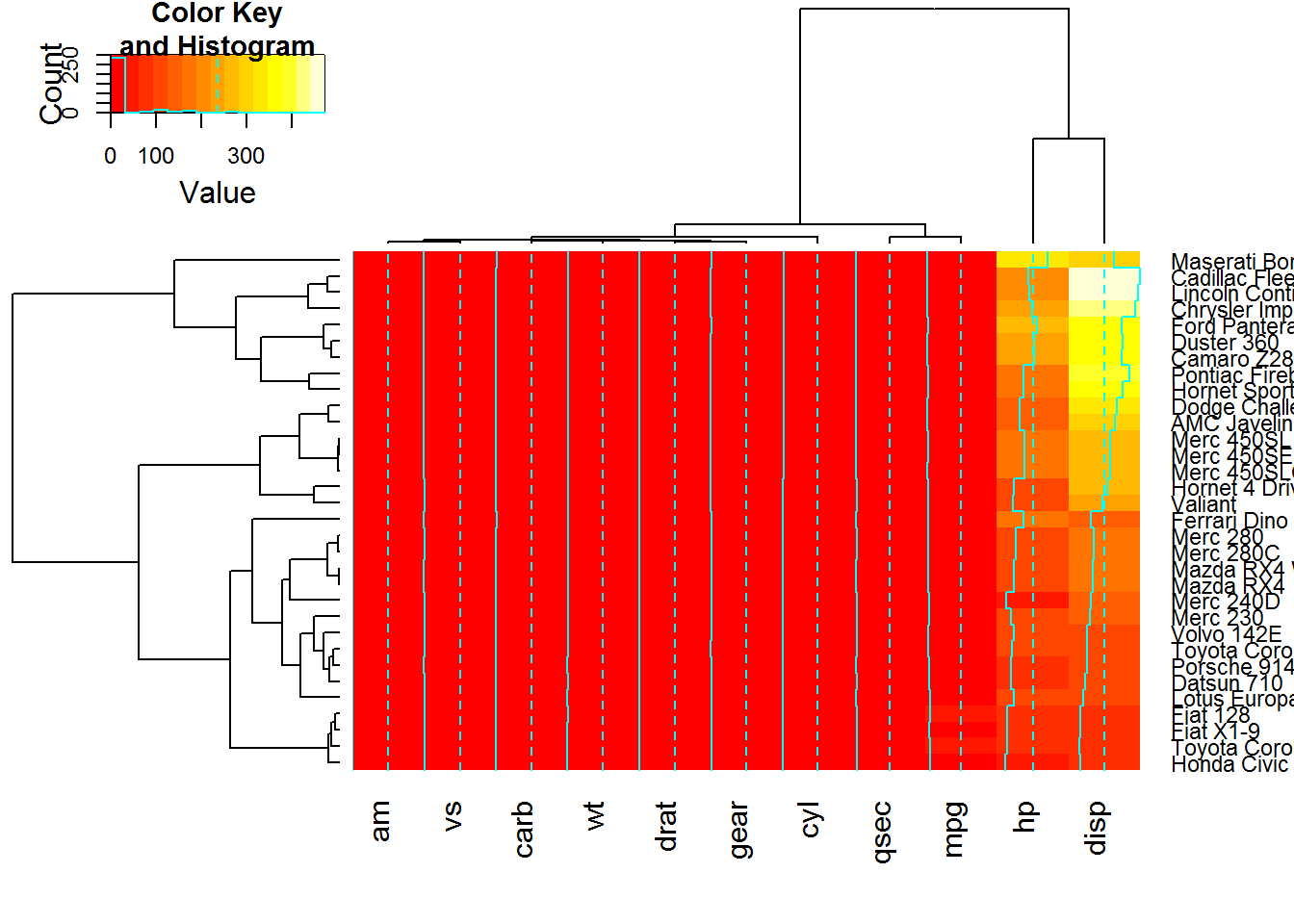``````## Show effect of row and column label rotation
heatmap.2(x, srtCol=NULL)````````heatmap.2(x, srtCol=0,   adjCol = c(0.5,1) )``